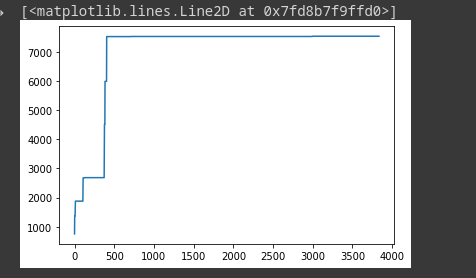# Memory usage increasing with number of steps while training

Hello
As I train the memory usage keeps increasing very rapidly as the number of steps increase.
I have also tried deleting all variables after every iteration.
Train loop:

``````def train_epoch(model: TransformerNetwork, optimizer):
global mem_used_arr_mbs
mem_used_arr_mbs = []
model.train()
losses = 0
train_iter = EnHindiDataset(split='train', language_pair=(SRC_LANGUAGE, TGT_LANGUAGE), limit=200_000)

mem_used = int(get_gpu_memory_usage().split())
mem_used_arr_mbs.append(mem_used)
src = src.to(DEVICE)
tgt = tgt.to(DEVICE)

tgt_input = tgt[:-1, :]

tgt_out = tgt[1:, :]
loss = loss_fn(logits.reshape(-1, logits.shape[-1]), tgt_out.reshape(-1))
loss.backward()

optimizer.step()
losses += loss.item()

del src, tgt, tgt_input
del logits, tgt_out, loss

``````Graph of memory usage vs n_steps. On x-axis are the steps and on y is the memory usage in mbs.
I don’t understand why the memory usage increases after each step, as pytorch don’t even need to store any information about the last step.

Memory consumption with time:After the epoch is some % complete I get this error: